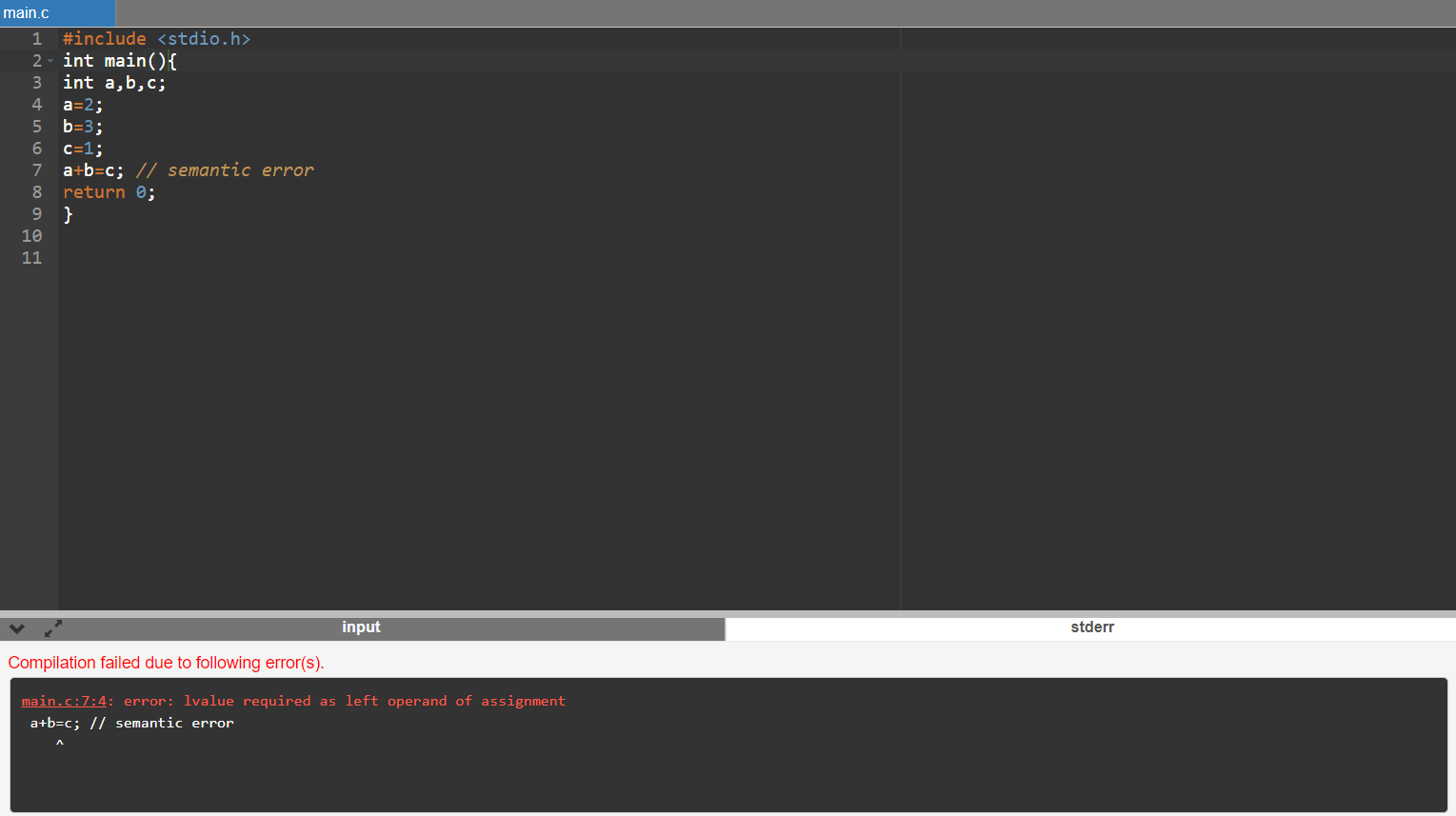# Program Errors and it's types

## A complete notes on program error and it's types for BCA and BIT students. What is program errors ? What is semantic error? What are the types of program error ? What is syntax error ? What is linker error ??Program Errors

Errors are the problems or the faults that occur in the program, which makes the behavior of the program abnormal, and experienced developers can also make these faults. Programming errors are also known as the bugs or faults, and the process of removing these bugs is known as debugging. These errors are detected either during the time of compilation or execution. Thus, the errors must be removed from the program for the successful execution of the program.

There are mainly five types of errors exist in C programming:

1. Syntax Error
2. Run time error
4. Logical error
5. Semantic error

Syntax error

Syntax errors are also known as the compilation errors as they occurred at the compilation time, or we can say that the syntax errors are thrown by the compilers. These errors are mainly occurred due to the mistakes while typing or do not follow the syntax of the specified programming language. These mistakes are generally made by beginners only because they are new to the language. These errors can be easily debugged or corrected.

Example:

Code:

#include <stdio.h>

int main()

{

printf("Hello World")

}

Output

Error] expected ';' before '}' token

Commonly occurred syntax errors are:

• If we miss the parenthesis ( } ) while writing the code.
• Displaying the value of a variable without its declaration.
• If we miss the semicolon (;) at the end of the statement.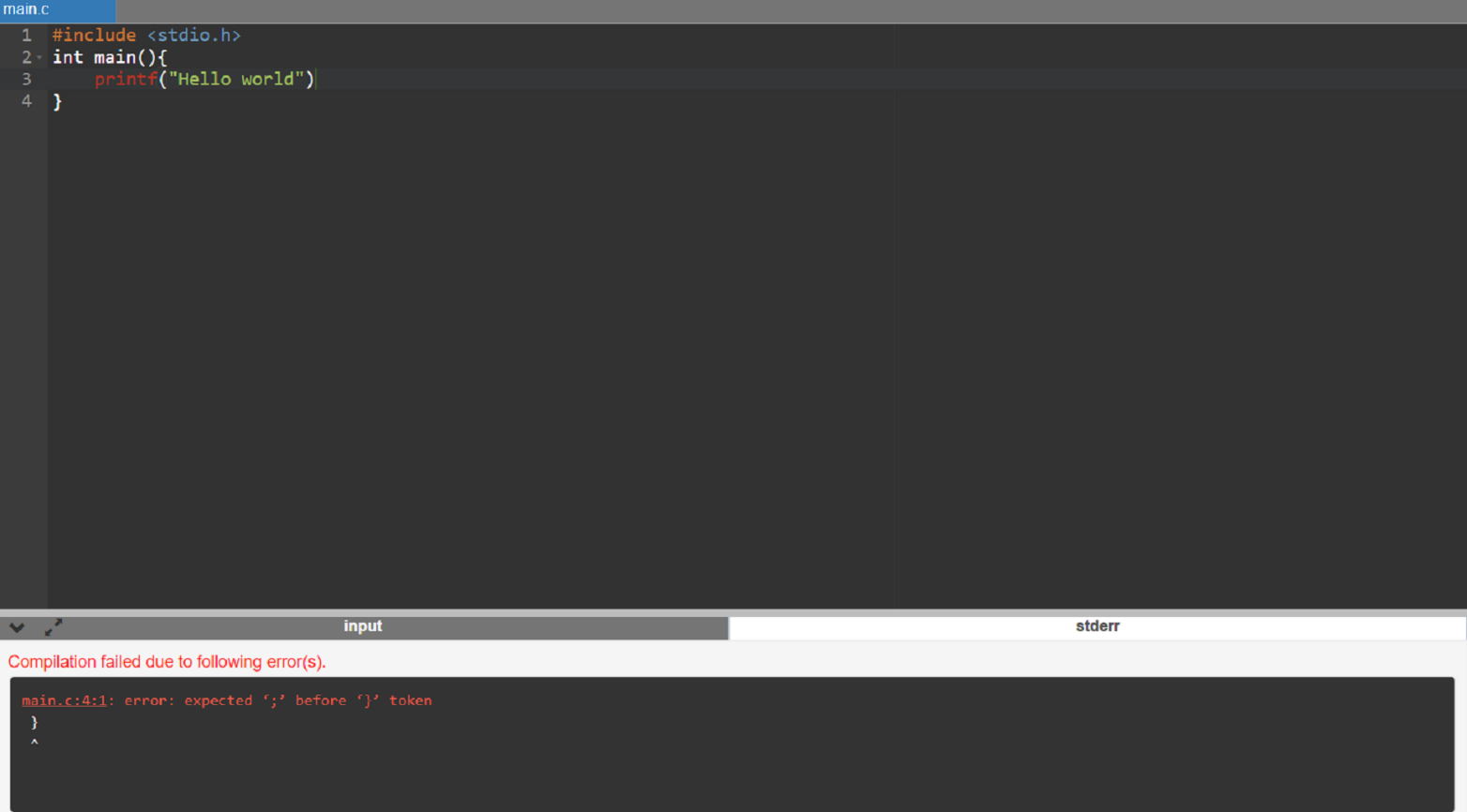Run time error

Sometimes the errors exist during the execution-time even after the successful compilation known as run-time errors. When the program is running, and it is not able to perform the operation is the main cause of the run-time error. The division by zero is the common example of the run-time error. These errors are very difficult to find, as the compiler does not point to these errors.

Example :

#include <stdio.h>

int main()

{

int a=2;

int b=2/0;

printf("The value of b is : %d", b);

return 0;

}

Output

Program crashes during runtime. In the above output, we observe that the code shows the run-time error, i.e., division by zero.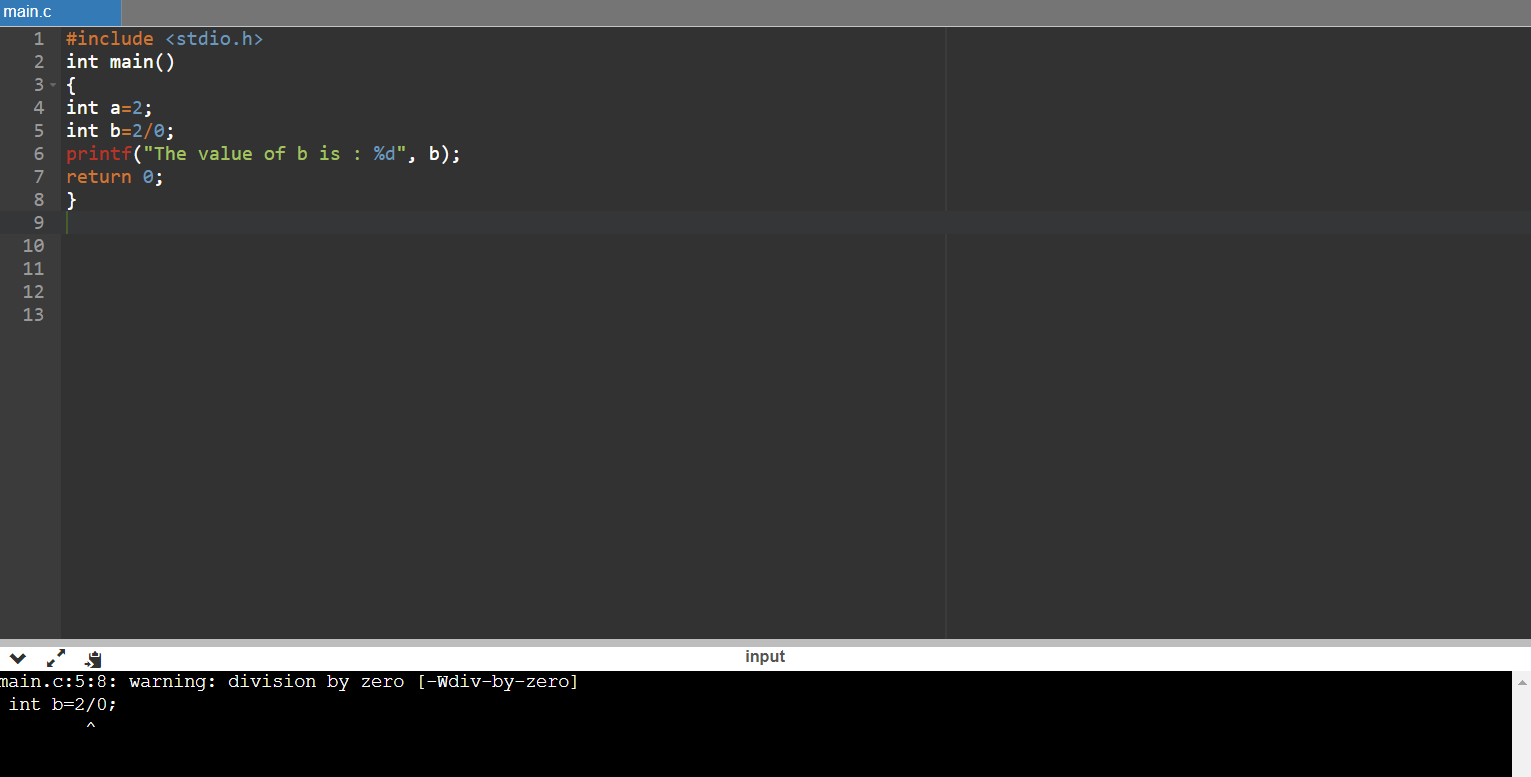This kind of errors are occurred, when the program is compiled successfully, and trying to link the different object file with the main object file. When this error is occurred, the executable is not generated, For example some wrong function prototyping, incorrect header file etc. If the main() is written as Main(), this will generate linked error.

Example:

Code

#include <stdio.h>

int Main()

{

int a=78;

printf("The value of a is : %d", a);

return 0;

}

Output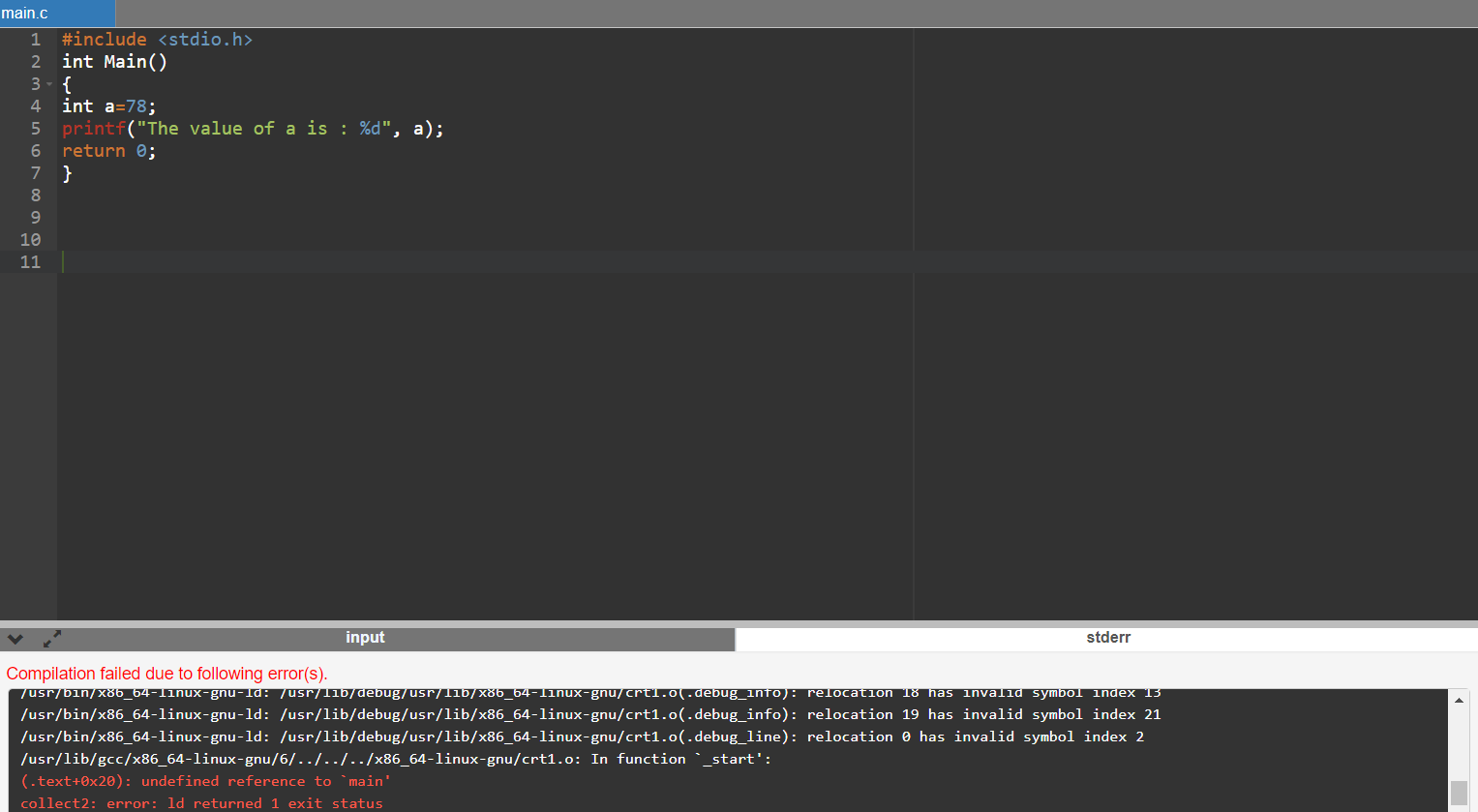Logical Error

The logical error is an error that leads to an undesired output. These errors produce the incorrect output, but they are error-free, known as logical errors. These types of mistakes are mainly done by beginners. The occurrence of these errors mainly depends upon the logical thinking of the developer. If the programmers sound logically good, then there will be fewer chances of these errors.

Example:

#include <stdio.h>

int main()

{

int sum=0; // variable initialization

int k=1;

for(int i=1;i<=10;i++); // logical error, as we put the semicolon after loop

{

sum=sum+k; k++;

}

printf("The value of sum is %d", sum);

return 0;

}

Output:

In the above code, we are trying to print the sum of 10 digits, but we got the wrong output as we put the semicolon (;) after the for loop, so the inner statements of the for loop will not execute. This produces the wrong output.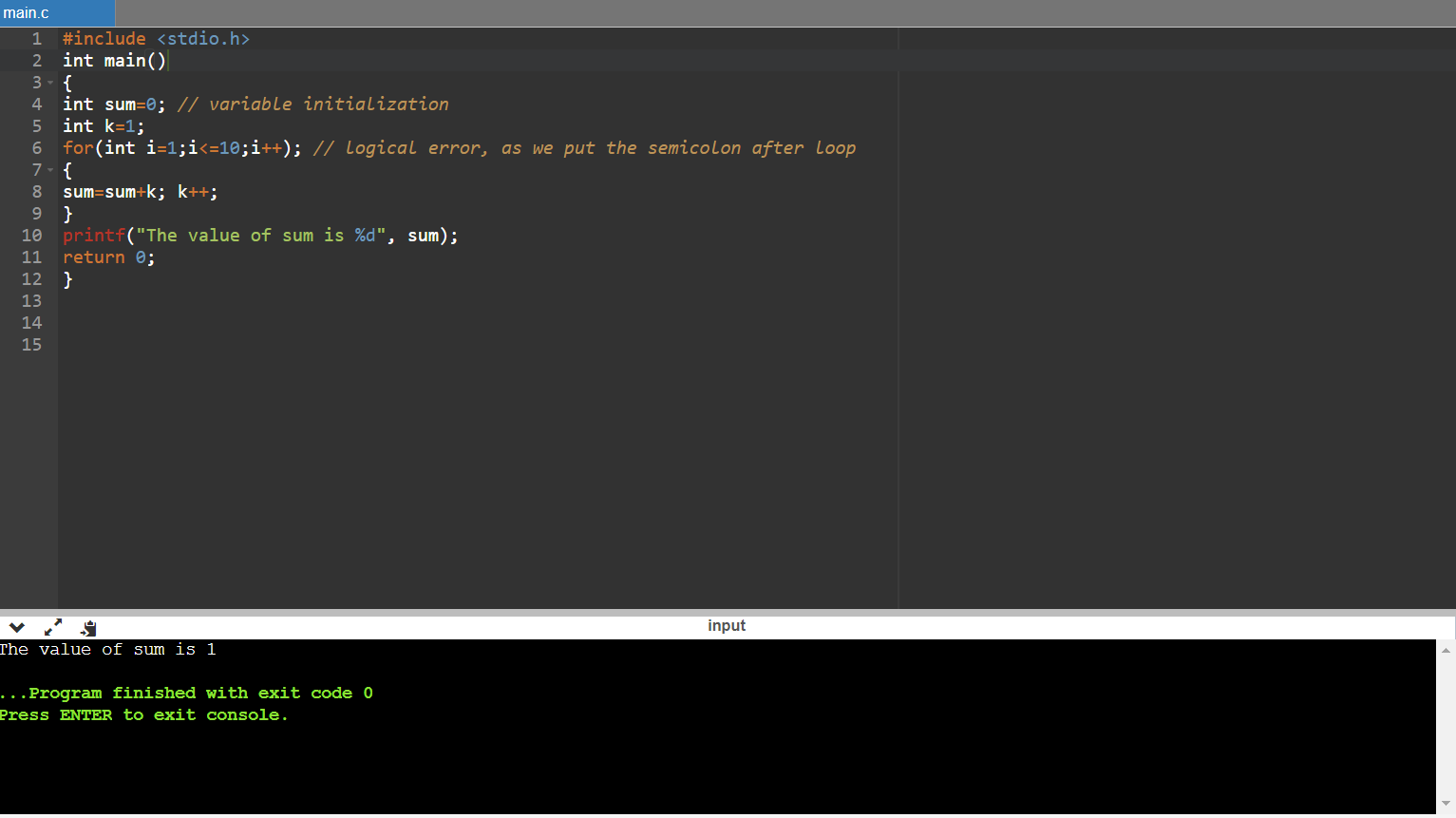Semantic Error

Semantic errors are the errors that occurred when the statements are not understandable by the compiler or it occurs due to an improper use of program statements.

The following can be the cases for the semantic error:

• Use of a un-initialized variable.

int i;

i=i+2;

• Type compatibility

int b = "javatpoint";

• Errors in expressions

int a, b, c;

a+b = c;

• Array index out of bound

int a;

a = 34;

Example:

Code

#include <stdio.h>

int main()

{

int a,b,c;

a=2;

b=3;

c=1;

a+b=c; // semantic error

return 0;

}

In the above code, we use the statement a+b =c, which is incorrect as we cannot use the two operands on the left-side.

Output: